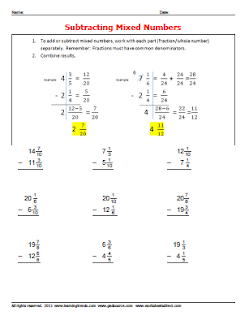## Tuesday, December 27, 2011

### Passing the GED Math Test

I often get calls from adult GED students wondering what they should study so that they can pass the GED Math Test. These are typically students who are looking to prepare at home with limited time and resources.  They may not have the means (vehicle) to get to a GED Study Center or the money to purchase a GED book.  They are ready to start but do not know where to begin.

I will first gently inform them that they need to have a good grasp on the basics of whole numbers, fractions, decimals, percents,  basic algebra, and basic geometry.  This alone can be daunting for some students because they may not even know their basic facts of adding, subtracting, multiplying, or dividing.

Next, I let them know that the GED math test is mostly application.  They will need to be able to read the GED Math problem, pull out important words and phrases, set up the problem, and solve it.  Many problems are multiple choice, but many will have you find the exact answer and then record it in a bubble chart.

I let them know that they are NOT alone when it comes to needing guidance in GED math preparation.  It has been my experience that most students benefit from at least some instruction.  Getting a good GED book from their local library, help from a friend, or instruction from a GED tutor/teacher will all help.

Finally, lets not forget about patience!  Students want to learn it and they want to learn it now!  And... when some learn new information, they may not be patient with themselves when it takes a bit longer to retain it.  Remind them that they may need to hear it more than once to remember it.... and that is OK!

## Saturday, November 26, 2011

### GED Math Vocabulary- Whole Numbers

Knowing and understanding the GED math vocabulary will give you GED math help when learning the basic concepts of whole numbers.  Download and print out free worksheets on whole number GED math vocabulary worksheets.  Worksheetsdirect is a great source for GED help!

## Tuesday, October 18, 2011

### Dividing Integers- Positive and Negative Numbers

Learning and practicing the "how to" of dividing integers and be difficult.  This link provides access to many dividing integers worksheets just like the one below.## Saturday, September 17, 2011

### GED Math Worksheets

I recently started a membership site for GED Math worksheets.  Check out www.worksheetsdirect.com

There are hundreds of worksheets...... just like the one below.  ﻿﻿www.worksheetsdirect.com

## Tuesday, March 29, 2011

Mean is the average of a group of numbers. To find the mean of a group of numbers you first add all the numbers together. You then divide by the number of elements you have in the data set. Let's try an example. Data set: 3, 5, 9, 8, 10 Number of elements: 5 Mean = (3 + 5 +9 + 8 + 10)/5 = 7 Now you can try an example. Data set: 6, 7, 8 11, 15, 7, 23 What is the data set? How many elements? What is the mean? Answer coming shortly.

## Saturday, January 01, 2011

GED Math Help: Simple Interest = PRT

The formula for finding simple interest is Principal x rate x time. One key concept is to remember that time is based on 1 year. Therefore, if the time is 5 years, you multiply by 5. However, if the time is 6 months, you multiply by 0.5 because 6 months is 1/2 of a year.

Lets try out this formula. Lets say that you are borrowing \$1000 for 3 years at 5% interest. How much interest would you earn?

I = 1000 * 0.05 * 3 You would earn \$150.00

Lets try another example. You are purchasing a car for \$12,500 and will be taking out a 5 year loan at 3%. What will be the TOTAL cost (car plus interest)?

12,500*0.03 * 5 = \$1875

To find the total cost, you should add the interest to the original principal.

\$12,500 + \$1875 = \$14375